Thank you for visiting nature.com. You are using a browser version with limited support for CSS. To obtain the best experience, we recommend you use a more up to date browser (or turn off compatibility mode in Internet Explorer). In the meantime, to ensure continued support, we are displaying the site without styles and JavaScript.

# Learning phase transitions by confusion

## Abstract

Classifying phases of matter is key to our understanding of many problems in physics. For quantum-mechanical systems in particular, the task can be daunting due to the exponentially large Hilbert space. With modern computing power and access to ever-larger data sets, classification problems are now routinely solved using machine-learning techniques1. Here, we propose a neural-network approach to finding phase transitions, based on the performance of a neural network after it is trained with data that are deliberately labelled incorrectly. We demonstrate the success of this method on the topological phase transition in the Kitaev chain2, the thermal phase transition in the classical Ising model3, and the many-body-localization transition in a disordered quantum spin chain4. Our method does not depend on order parameters, knowledge of the topological content of the phases, or any other specifics of the transition at hand. It therefore paves the way to the development of a generic tool for identifying unexplored phase transitions.

## Main

Machine learning as a tool for analysing data is becoming more and more prevalent in an increasing number of fields. This is due to a combination of availability of large amounts of data and the advances in hardware and computational power, the latter most notably through the use of graphical processing units.

Two typical methods of machine learning can be distinguished, namely the unsupervised and supervised methods. In the former the machine receives no input other than the data and is asked, for example, to extract features or to cluster the samples. Such an unsupervised approach was applied to identify phase transitions and order parameters from images of classical configurations of Ising models5. In the supervised learning methods, the data have to be supplemented by a set of labels. A typical example is classification of data, where each sample is assigned a class label. The machine is trained to recognize samples and predict their associated label, demonstrating that it has learned by generalizing to samples it has not encountered before. This approach, too, has been demonstrated on Ising models6.

Concepts from physics have also found their way into the field of machine learning. Examples of this are the relations between neural networks (NNs) and statistical Ising models and renormalization flow7, the use of tensor network techniques to train them8, using reinforcement learning to make networks represent wavefunctions9, and indeed the very concept of phase transitions themselves10.

Motivated by previous studies, we apply machine-learning techniques to the detection of phase transitions. In contrast to the earlier works, however, we focus on a combination of supervised and unsupervised techniques. In most cases, namely, it is exactly the labelling that one would like to find out (that is, classification of phases). That implies that a labelling is not known beforehand, and hence supervised techniques are not directly applicable. In this Letter we demonstrate that it is possible to find the correct labels, by purposefully mislabelling the data and evaluating the performance of the machine learner. We will base our method on NNs, which are capable of fitting arbitrary nonlinear functions11. Indeed, if a linear feature extraction method worked, there would have been no need to explicitly find labels in the first place.

We emphasize the main result in this work is that with the proposed method we are able to find a consistent labelling for data that have distinct patterns. A change in the pattern of some observable is not necessarily correlated with a physical phase transition. Our method is capable of recognizing the change of pattern, after which it is up to the user to investigate whether the change corresponds to a crossover or a phase transition. We remark that we do not exclude the possibility that linear methods would be able to perform some of the tasks we describe below. Nor do we exclude the possibility that other methods such as latent-variable models or other maximum likelihood algorithms would be able to perform the same task. Finding the correct method or transformation of the data may be a prohibitive task however, and so using a (possibly overpowered) method such as NNs provides a useful starting point. Our method boils down to bootstrapping a supervised learning method to an unsupervised one, at the expense of computational time.

Additionally, but not less important, we propose the use of the entanglement spectrum (ES; to be defined below) as the input data on which to detect patterns and phase transitions. This allows for the novelty of studying quantum models instead of classical models as was done in previous literature. In the following we explain and demonstrate our method on two quantum-mechanical models and on the classical Ising model.

For quantum phase transitions, one tries to learn the quantum-mechanical wavefunction |ψ〉, which contains exponentially many coefficients with increasing system size. As has been noted before6, a similar problem exists in the field of machine learning: the number of samples in a data set has to increase exponentially with the number of features one is trying to extract. To prevent having to deal with exponentially large wavefunctions, we pre-process the data in the form of the ES12, which has been shown to contain important information about |ψ〉 (refs 13,14).

To justify the use of the ES, we note that recently the quantum entanglement has taken up a major role in the characterization of many-body quantum systems13,15. In particular, the ES has been used as an important tool in, for example, fingerprinting topological order16,17,18, tensor network properties19,20, quantum critical points, symmetry-breaking phases21,22, and even many-body localization23,24. Very recently, an experimental protocol for measuring the ES has been proposed25. On the level of the ES, the information of phases is not clearly identifiable as in the classical images, which we will show in the following sections. However, patterns in the ES suggest that learning and generalization is still possible.

We will next consider the Kitaev chain as a demonstration of our method. The Kitaev chain serves as an excellent example since analytical results are available, and the ES shows a clear distinction between the two phases of the model. We demonstrate the generalizing power of the NN by blanking out the training data around the transition, and show that it can still predict the transition accurately. We then purposefully mislabel the data, thereby confusing the network, and introduce the characteristic shape of the networks’ performance function.

The Kitaev chain model is defined through the following Hamiltonian: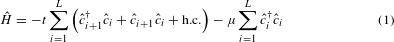where t > 0 controls the hopping and the pairing of spinless fermions alike and μ is a chemical potential. The ground state of this model has a quantum phase transition from a topologically trivial (|μ| > 2t) to a non-trivial state (|μ| < 2t) as the chemical potential μ is tuned across μ = ±2t.

We use the ES to compress the quantum-mechanical wavefunction. The ES is defined as follows. The whole system is first divided into two subsets A and B, after which the reduced density matrix of subset A is calculated by partially tracing out the degrees of freedom in B, that is, ρA = TrB |ψ〉〈ψ|. Denoting the eigenvalues of ρA as λi, the ES is then defined as the set of numbers −lnλi. It is important to remark that various types of bipartition of the whole system into subsets A and B exist, such as dividing the bulk into extensive disconnected parts26, divisions in momentum space27 or indeed even random partitioning28. In this work, we use the usual spatial bipartition into left and right halves of the whole system.

As shown in Fig. 1a, the ES of the Kitaev chain is clearly distinguishable in the two phases, especially since the non-trivial phase has a degeneracy structure as do all symmetry-protected topological phases18. This feature is clear also for human eyes, and a machine-learning routine is overkill. We use this model for demonstration purposes and in the following, we will apply the introduced methodology to more complex models. The data for machine learning are chosen to be the largest 10 eigenvalues λi, for L = 20 with an equal partitioning LA = LB = 10, and for various values of −4tμ ≤ 0.Figure 1: Learning the topological phase transition in the Kitaev chain.

First we perform unsupervised learning, using an established method for feature extraction. The entanglement spectra are interpreted as points in a 10-dimensional space, and we use principal component analysis (PCA)29 to extract mutually orthogonal axes along which most of the variance of the data can be observed. PCA amounts to a linear transformation Y = XW, where X is an N × 10 matrix containing the entanglement spectra as rows (N = 104 is the number of samples).

The orthogonal matrix W has vectors representing the principal components ω as its columns, which are determined through the eigenvalue equation XT = λω. The eigenvalues λ are the singular values of the matrix X, and are hence non-negative real numbers, and we normalize them such that ∑λ = 1. The result of PCA is shown in Fig. 1b, and it is indeed possible to cluster the spectra into three sets: μ < −2t, μ = −2t and μ > −2t.

We now turn to training a feedforward NN on the 10-dimensional inputs, and refer to the online Methods and ref. 30 for more details. For completeness, we mention the essentials of NNs in Fig. 2.

We train the network with 80 hidden sigmoid neurons in a single hidden layer, and 2 output neurons. The first/second output neuron predicts the (not necessarily normalized) probability for the data to be in trivial/non-trivial phase, and the predicted phase is the phase with the larger probability. We use stochastic gradient descent and l2 regularization to try to minimize a cross-entropy cost function. The network easily learns to distinguish the spectra and is able to generalize to unseen data points.

Arguably the most important objective of machine learning in general is that of generalization. After all, learning is demonstrated by being able to perform well on examples that have not been encountered before. As another display of the generalizing power of the network, we blank out the data in a width w around μ = −2t and ask the network to interpolate and find the transition point. Figure 1c shows that the network has no difficulties doing so even for w = 2t. We were able to go up to widths w = 3t before training became unreliable.

The PCA as an unsupervised learning technique may be applied without perfectly known information of the system, but it is a linear analysis and is hence incapable of extracting nonlinear relationships among the data. On the other hand, a NN is capable of fitting any nonlinear function11, but a training phase with correctly labelled input–output pairs is needed. In the following, we propose a scheme combining both supervised and unsupervised methods that we refer to as a confusion scheme. This scheme is the main result of this work.

We suppose that the data depend on a parameter that lies in the range (a, b), and we assume that there exists a critical point a < c < b such that the data can be classified into two groups. However, we do not know the value of c. We propose a critical point c′, and train a network that we call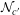by labelling all data with parameters smaller than c′ with label 0 and the others with label 1. Next, we evaluate the performance ofon the entire data set and refer to its total performance, with respect to the proposed critical point c′, as P(c′). We will show that the function P(c′) has a universal W-shape, with the middle peak at the correct critical point c. Applying this to the Kitaev model, we can see from Fig. 1d that for −4t < μ < 0, the prediction performance from the confusion scheme has a W-shape with the middle peak at μ = −2t.

The W-shape can be understood as follows. We assume that the data have two different structures in the regimes below c and above c, and that the NN is able to find and distinguish them. We refer to these different structures as features. When we set c′ = a, the NN chooses to assign label 1 to both features and thus correctly predicts 100% of the data. A similar analysis applies to c′ = b, except that every data point is assigned the label 0. When c′ = c is the correct labelling, the NN will choose to assign the right label to both sides of the critical point and again performs perfectly. When a < c′ < c, in the training phase the NN sees data with the same feature in the ranges from a to c′ and from c′ to c, but having different labels (hence the confusion). In this case it will choose to learn the label of the majority data, and the performance will be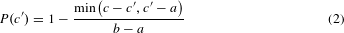Similar analysis applies to c < c′ < b. This gives the typical W-shape seen in Fig. 1d. Note that if the point c is not exactly centred between a and b, the W-shape will be slightly distorted. Its middle peak always corresponds to the correct labelling, but the depth of the minima will differ between the left and right.

We test the confusion scheme on the thermal phase transition in the two-dimensional classical Ising model, which has been studied by both supervised learning6 and unsupervised learning5 methods. Here we train a NN (with L2 neurons in the input and hidden layers, and 2 neurons in the output layer) on the L × L classical configurations sampled from Monte Carlo simulations. As shown in Fig. 3, the W-shape again predicts the right transition temperature. Note the confusion scheme works better when the underlying feature in the data is sharper, that is, for the larger system size L = 20. We also remark that the error bars shown in the figure are large for the points deviating from the expected W-shape. These error bars were obtained by repeating the confusion procedure with Monte Carlo data from independent runs.

To confirm that the confusion scheme indeed extracts non-trivial features from the input data, we have checked the performance curve from the confusion scheme, when the NN is trained on unstructured random data. We use a fictive parameter as a tuning parameter, but have completely unstructured (random) data as a function of it. Hence, the network will not find structure in the data, and a correct labelling does not exist. The middle peak of the characteristic W-shape disappears, turning it into a V-shape.

We will now test our proposed scheme on an example where the exact location of the transition point is not known. We study a case of interest in recent literature, namely that of many-body localization. We consider the following model: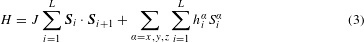where S denote spin-1/2 operators. The local fields hiα are drawn from a uniform box distribution with zero mean and width hmaxα. We set hmaxx = hmaxz = hmax and hmaxy = 0. The disorder allows us to generate many samples at a fixed set of model parameters, in analogy to the different configurations for a fixed temperature in the classical spin systems5,6.

The model in equation (3) has a transition between thermalizing and non-thermalizing (that is, many-body localized) behaviour, driven by the disorder strength hmax. In particular, when varying hmax, both the energy level statistics as well as the statistics of the entanglement spectra change their nature24. For the case of the energy levels, the gaps (level spacings) follow either a Wigner–Dyson distribution for the thermalizing phase, or a Poisson distribution for the localized phase; while for the ES, theWigner–Dyson distribution is replaced by a semi-Poisson distribution. Note that the change of ES can already be seen from the statistics in a single eigenstate24.

We numerically obtain the ES for the ground state of the model in equation (3), for disorder strengths between hmax = J and hmax = 5J. The transition was shown to happen around hmax 3J (ref. 24), but we stress that our method does not rely on this knowledge. We would simply have started from a larger width of points, and then systematically narrow it down to the current range. At each value of hmax we generate 105 disorder realizations for system size L = 12 and calculate the ES for LA = LB = 6. These 26 = 64 levels are used as the input to the NN.

First, we try to use an unsupervised PCA to cluster the data. This analysis shows that the first two principal components are dominant, with the other components being of order 10−4 or less. However, a scatterplot of the data when projected onto the first two principal components (shown in Fig. 4a) does not reveal a clear clustering of the spectra.

We therefore turn to train a shallow feedforward network on the entanglement spectra to use the confusion scheme. Here we use a network with 64 input neurons, 100 hidden neurons and 2 output neurons. The results are shown in Fig. 4b. Also in this case, the characteristic W-shape is obtained and we detect the transition at hc 3J. In addition to the previous cases, we also consider explicitly the performance of the network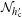at hc′. We do this to confirm that the labelling with hc′ at 3J is indeed correct. We expect that the training of the network is most robust against changes in its parameters for the correct labelling. In other words, we may also look for the hc′ at which the training is most independent of chosen conditions. As shown in Fig. 4c, this point is also at hc.

An interesting direction for future studies is the relaxation of the assumption that there are only two phases to be distinguished. If there are multiple phase transitions present in the data as a function of the tuning parameter, the characteristic W-shape will be modified, and its new shape (that is, the number of peaks) will signal the correct number of different labels. This is due to the fact that data with multiple phases can always be bipartitioned into classes ‘belongs to phase A’ and ‘does not belong to phase A’, where A can be any phase in the data. Additionally, it may be possible to formulate this method in a self-consistent way, with an adaptive labelling and having the algorithm determine the correct labels by itself.

## Methods

In this section we will describe in detail the method of NNs. A more extensive pedagogical introduction can be found in ref. 30. To do so, we first introduce the concept of an artificial neuron, as depicted in Fig. 2a in the main text. The artificial neuron we consider has a number of n inputs, and a single output. To each of the inputs is associated an incoming value xi and a weight wi, i = 1…n from which the neuron computes its output y. This is done according to y = f(a) with a being the weighted sum of the inputs, that is, a = ∑iwixi, and f(.) representing an activation function. A typical choice for the activation function (and indeed the one we have used) is the sigmoid f(a) = 1/(1 + ea), turning our artificial neuron into a sigmoid neuron. We also mention the common RELU neuron (rectified linear unit), for which the activation function reads f(a) = (a) with Θ(a) representing the Heaviside step function.

From a single neuron we are now able to construct a so-called feedforward NN, by combining layers of neurons as shown in Fig. 2b in the main text. Such a network consists of layers (represented as columns in the figure) of neurons, whose outputs are fed into the next layer as inputs. Two points here must be remarked upon. First, although each neuron is shown to have many outgoing connections as opposed to the neuron we just introduced, each of these is assigned the same outgoing value. Second, the neurons in the first layer (column) of the network, called the input layer, have no incoming values but instead are ‘dummy’ neurons whose outputs are assigned the values of the input data. There can be arbitrarily many ‘hidden’ layers, each with an arbitrary number of neurons, until we reach the final output layer. The connections between neurons in layer i and i + 1 are associated with a weight matrix w[i], such that wnm[i] is the weight between neuron n in layer i and neuron m in layer i + 1. We will be concerned with networks that have a single hidden layer, falling under the class of shallow networks, as opposed to deep learning networks consisting of multiple layers.

At this point, the network provides a black-box function g(x; W) that provides the predicted output of the network for a given input x, and depends on all of the weights W = {w, …, w[n−1]} between the neurons. This output is a vector of length equal to the number of neurons in the output layer. Having a single output is equivalent to doing a type of regression, whereas here we will mostly use two outputs as we will describe below. The training of the network now proceeds iteratively as follows. The weights are initialized randomly at first, after which we start feeding input samples through the network. For the sake of simplicity, denoting the output of the network by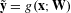, we seek to change the weights such that we minimize the cost function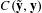, with y representing the correct (targeted) output corresponding to input x. Typical cost functions used in the literature are the quadratic-cost function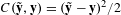and the cross-entropy cost function defined as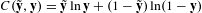. We have chosen to work with the latter. The optimization of the weights is done via the standard backpropagation algorithm, which is in essence gradient descent on the function g(x; W). This updates the weights iteratively such that WW + αΔW, with α being a parameter called the learning rate. We also mention that instead of feeding through single samples to compute the gradient, we may use a batch of inputs of size Nb to compute the average gradient for faster convergence.

To prevent the network from overfitting the data, we include a standard l2 regularization term. This term enters the cost function as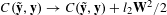, such that using gradient descent we try to keep the weights small when l2 > 0.

We note that the choice of the learning rate (α) and regularization (l2) is essential for a successful training. The use of regularization is expected to reduce overfitting and make the network less sensitive to small variations of the data, hence forcing it to learn its structure. However, the confusion scheme of the main text depends solely on the ability of finding the majority label for the underlying structure in the data. In this sense, overfitting is not necessarily bad. Indeed, we have observed that training with a negative l2 may lead to an equally good performance. We speculate that this is because a negative l2 tries to quickly increase the weights, making it harder for the network to change its opinion about data samples in later stages. If the initial training data are uniformly sampled, meaning the majority data are indeed represented by a majority, the network will rapidly adjust its weights to this majority. The training is stopped when a clear W-shape is formed.

For the quantum models, the input to the NN is the ES, which has the nice property that successive singular values decay very fast. Thus, we have kept a fixed number of singular values and the computational time is independent of the system size. For the classical models, the input is the classical configuration. In this case we fix the number of hidden neurons and increase the numbers of input neurons according to the system size N, thus the complexity is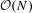.

Last we mention the absence of error bars. Obtaining error bars as is typically done by averaging over different disorder realizations is not feasible, since the performance of the network is itself already an average over such realizations. Instead, we might train different networks with different initial weights and average over those, so that we obtain an averaged W-shape. However, the error bars thus obtained do not shed light on the location of the transition. Once a W-shape is identified in the training, one may instead tweak the network parameters to optimize the shape.

### Data availability.

The data that support the plots within this paper and other findings of this study are available from the corresponding author on request.

## References

1. 1

Jordan, M. I. & Mitchell, T. M. Machine learning: Trends, perspectives, and prospects. Science 349, 255–260 (2015).

2. 2

Kitaev, A. Y. Unpaired majorana fermions in quantum wires. Phys.-Usp. 44, 131 (2001).

3. 3

Onsager, L. Crystal statistics. I. A two-dimensional model with an order-disorder transition. Phys. Rev. 65, 117–149 (1944).

4. 4

Nandkishore, R. & Huse, D. A. Many-body localization and thermalization in quantum statistical mechanics. Annu. Rev. Condens. Matter Phys. 6, 15–38 (2015).

5. 5

Wang, L. Discovering phase transitions with unsupervised learning. Phys. Rev. B 94, 195105 (2016).

6. 6

Carrasquilla, J. & Melko, R. G. Machine learning phases of matter. Nat. Phys. http://dx.doi.org/10.1038/nphys4035 (2017).

7. 7

Mehta, P. & Schwab, D. J. An exact mapping between the variational renormalization group and deep learning. Preprint at http://arxiv.org/abs/1410.3831 (2014).

8. 8

Stoudenmire, E. M. & Schwab, D. J. Supervised learning with quantum-inspired tensor networks. Preprint at https://arxiv.org/abs/1605.05775 (2016).

9. 9

Carleo, G. & Troyer, M. Solving the quantum many-body problem with articial neural networks. Preprint at https://arxiv.org/abs/1606.02318 (2016).

10. 10

Saitta, L. & Sebag, M. Encyclopedia of Machine Learning 767–773 (Springer, 2010).

11. 11

Haykin, S. O. Neural Networks: A Comprehensive Foundation (Prentice Hall, 1998).

12. 12

Li, H. & Haldane, F. D. M. Entanglement spectrum as a generalization of entanglement entropy: identification of topological order in non-abelian fractional quantum Hall effect states. Phys. Rev. Lett. 101, 010504 (2008).

13. 13

Laflorencie, N. Quantum entanglement in condensed matter systems. Phys. Rep. 646, 1–59 (2016).

14. 14

Chandran, A., Khemani, V. & Sondhi, S. L. How universal is the entanglement spectrum? Phys. Rev. Lett. 113, 060501 (2014).

15. 15

Amico, L., Fazio, R., Osterloh, A. & Vedral, V. Entanglement in many-body systems. Rev. Mod. Phys. 80, 517–576 (2008).

16. 16

Thomale, R., Sterdyniak, A., Regnault, N. & Bernevig, B. A. Entanglement gap and a new principle of adiabatic continuity. Phys. Rev. Lett. 104, 180502 (2010).

17. 17

Qi, X. L., Katsura, H. & Ludwig, A. W. W. General relationship between the entanglement spectrum and the edge state spectrum of topological quantum states. Phys. Rev. Lett. 108, 1–5 (2012).

18. 18

Turner, A. M., Pollmann, F. & Berg, E. Topological phases of one-dimensional fermions: an entanglement point of view. Phys. Rev. B 83, 075102 (2011).

19. 19

Cirac, J. I., Poilblanc, D., Schuch, N. & Verstraete, F. Entanglement spectrum and boundary theories with projected entangled-pair states. Phys. Rev. B 83, 245134 (2011).

20. 20

Schuch, N., Poilblanc, D., Cirac, J. I. & Pérez-García, D. Topological order in the projected entangled-pair states formalism: transfer operator and boundary hamiltonians. Phys. Rev. Lett. 111, 090501 (2013).

21. 21

Calabrese, P. & Lefevre, A. Entanglement spectrum in one-dimensional systems. Phys. Rev. A 78, 032329 (2008).

22. 22

Alba, V., Haque, M. & Läuchli, A. M. Boundary-locality and perturbative structure of entanglement spectra in gapped systems. Phys. Rev. Lett. 108, 227201 (2012).

23. 23

Yang, Z.-C., Chamon, C., Hamma, A. & Mucciolo, E. R. Two-component structure in the entanglement spectrum of highly excited states. Phys. Rev. Lett. 115, 267206 (2015).

24. 24

Geraedts, S. D., Nandkishore, R. & Regnault, N. Many-body localization and thermalization: insights from the entanglement spectrum. Phys. Rev. B 93, 174202 (2016).

25. 25

Pichler, H., Zhu, G., Seif, A., Zoller, P. & Hafezi, M. Measurement protocol for the entanglement spectrum of cold atoms. Phys. Rev. X 6, 041033 (2016).

26. 26

Hsieh, T. H. & Fu, L. Bulk entanglement spectrum reveals quantum criticality within a topological state. Phys. Rev. Lett. 113, 106801 (2014).

27. 27

Thomale, R., Arovas, D. P. & Bernevig, B. A. Nonlocal order in gapless systems: entanglement spectrum in spin chains. Phys. Rev. Lett. 105, 116805 (2010).

28. 28

Vijay, S. & Fu, L. Entanglement spectrum of a random partition: connection with the localization transition. Phys. Rev. B 91, 220101 (2015).

29. 29

Pearson, F. K. On lines and planes of closest fit to systems of points in space. Philos. Mag. 2, 559–572 (1901).

30. 30

Nielsen, M. Neural Networks and Deep Learning (Determination Press, 2015).

## Acknowledgements

E.P.L.v.N. and S.D.H. gratefully acknowledge financial support from the Swiss National Science Foundation (SNSF). Y.-H.L. is supported by ERC Advanced Grant SIMCOFE. E.P.L.v.N. acknowledges fruitful discussions with M. Koch-Janusz on extending the confusion scheme to the case with multiple phases. E.P.L.v.N. and Y.-H.L. acknowledge helpful discussions with G. Carleo, J. Osorio and L. Wang. E.P.L.v.N. and S.D.H. thank A. Krause for useful discussion on machine learning.

## Author information

Authors

### Contributions

E.P.L.v.N. and Y.-H.L. conceived the ideas; S.D.H. supervised the research. All authors contributed to the writing of the manuscript.

### Corresponding author

Correspondence to Evert P. L. van Nieuwenburg.

## Ethics declarations

### Competing interests

The authors declare no competing financial interests.

## Rights and permissions

Reprints and Permissions

• ### Revisiting the dynamics of Bose-Einstein condensates in a double well by deep learning with a hybrid network

• Shurui Li
• Jianqin Xu
• Weiping Zhang

Frontiers of Physics (2022)

• ### Quantum cluster algorithm for data classification

• Junxu Li
• Sabre Kais

Materials Theory (2021)

• ### Neural network-based order parameter for phase transitions and its applications in high-entropy alloys

• Junqi Yin
• Zongrui Pei
• Michael C. Gao

Nature Computational Science (2021)

• ### Learning spin liquids on a honeycomb lattice with artificial neural networks

• Chang-Xiao Li
• Sheng Yang
• Jing-Bo Xu

Scientific Reports (2021)

• ### Estimating the effective fields of spin configurations using a deep learning technique

• D. B. Lee
• H. G. Yoon
• C. Won

Scientific Reports (2021)

## Search